Next: Triangulations of Up: Order vs. Algebraic Topology Previous: Cutsets

## Truncated Lattices

One of the most intriguing results in the fixed point theory of ordered sets is Baclawski and Björner's result that every   finite truncated noncomplemented lattice has the fixed point property. The original proof (cf. , Corollary 3.2) is quite complex (it ultimately goes back to the intricate topological arguments in , Theorem 3.2, resp. to the arguments in , Corollary 6.3) and seems to be not algorithmic. The reduction arguments of Constantin and Fournier can be used to give another proof of this result. Interestingly even though Baclawski and Björner's result is topological and should thus have analogues for the fixed simplex and the fixed clique property, we need to assume that we are working with ordered sets for this proof.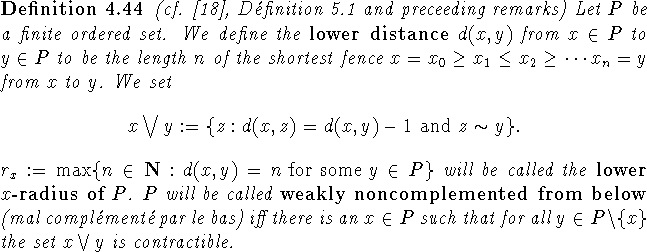Baclawski and Björner's classical result now easily follows from: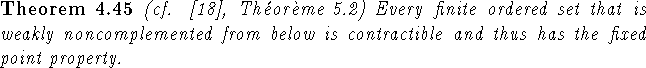Proof: The proof is an induction on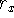, the lower x-radius of P, with x as in the definition of weak noncomplementedness from below. For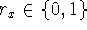there is nothing to prove as P has a largest element. Now assume that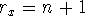and the theorem holds for all weakly noncomplemented from below sets P with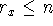. Then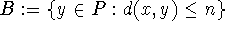is weakly noncomplemented from below and thus contractible. It is thus sufficient to verify that the conditions of Theorem 4.41 are satisfied. (This is again a nice exercise in the translations between ordered sets, graphs and simplicial complexes.) We can assume without loss of generality that n is even.
Let C be a chain in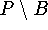and let y be the largest element of C. Then there is a fence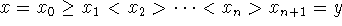from x to y. We will now show that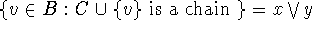, which is contractible, thus concluding the proof: Let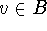be such that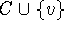is a chain. Then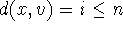. Moreover since v is comparable to y we infer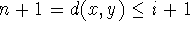. Thus d(x,v)=n and thus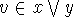. Conversely if, then d(x,v)=n, so. Moreover we must have v> y (otherwise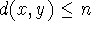), which means thatis a chain. \Next: Triangulations of Up: Order vs. Algebraic Topology Previous: Cutsets

Bernd.S.W.Schroder